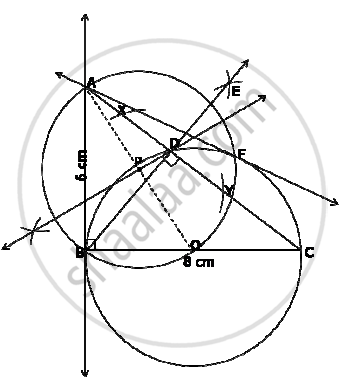# Construct a right triangle ABC with AB = 6 cm, BC = 8 cm and ∠B = 90°. Draw BD, the perpendicular from B on AC. Draw the circle through B, C and D and construct the tangents from A to this circle. - Mathematics

Construct a right triangle ABC with AB = 6 cm, BC = 8 cm and ∠B = 90°. Draw BD, the perpendicular from B on AC. Draw the circle through B, C and D and construct the tangents from A to this circle.

#### Solution

Steps of construction:
1) Construct the triangle as per given measurements.
2) Take any arbitrary radius and draw two arcs of circle from point B on AC, intersecting AC at X and Y.
3) Taking X and Y as centres, draw two arcs of circles to intersect each other at point E. Join B and E. BE is the perpendicular from B on AC.
4) DBDC is a right angled. Hence, BC the hypotenuse will form the diameter of the circle passing through the vertices of ΔBDC.
5) BC = 8 cm OC = 4 cm. draw a circle of radius equal 4 cm, passing through B, D and C.
6) Join O and A. Obtain the mid-point P of segment OA by drawing perpendicular bisector to OA.
7) Draw a circle with centre P and radius AP.
8) Let B and F be the points of intersection of these two circles. Hence, AB and AF are the required tangents.Concept: Constructions Examples and Solutions
Is there an error in this question or solution?
2014-2015 (March) All India Set 2

Share